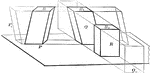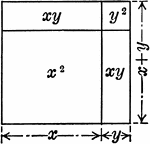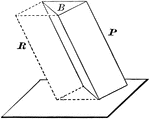Volume of Parallelopiped

Diagram used to prove the theorem: "The volume of a any parallelopiped is equal to the product of its…Representation of Algebraic Product of (x+y)(x+y)

An geometric illustration of the algebraic product of (x+y)(x+y).Volume of Triangular Prism

Diagram used to prove the theorem: "The volume of a triangular prism is equal to the product of its…